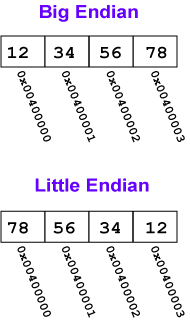Any integer that is a multiple of 4 looks like `4*N` for some `N`.

How can you multiply by four in binary? By shifting left 2 positions. So a multiple of 4 looks like some `N` shifted left two positions. So the low order two bits of a multiple of 4 are both 0.

• `0x000AE430   Yes.`
• `0x00014432   No.`
• `0x000B0737   No.`
• `0x0E0D8844   Yes.`

# Big Endian and Little EndianA load word or store word instruction uses only one memory address. The lowest address of the four bytes is used for the address of a block of four contiguous bytes.

How is a 32-bit pattern held in the four bytes of memory? There are 32 bits in the four bytes and 32 bits in the pattern, but a choice has to be made about which byte of memory gets what part of the pattern. There are two ways that computers commonly do this:

Big Endian Byte Order: The most significant byte (the "big end") of the data is placed at the byte with the lowest address. The rest of the data is placed in order in the next three bytes in memory.

Little Endian Byte Order: The least significant byte (the "little end") of the data is placed at the byte with the lowest address. The rest of the data is placed in order in the next three bytes in memory.

In these definitions, the data, a 32-bit pattern, is regarded as a 32-bit unsigned integer. The "most significant" byte is the one for the largest powers of two: 231, ..., 224. The "least significant" byte is the one for the smallest powers of two: 27, ..., 20.

For example, say that the 32-bit pattern 0x12345678 is stored at address 0x00400000. The most significant byte is 0x12; the least significant is 0x78.

Within a byte the order of the bits is the same for all computers (no matter how the bytes themselves are arranged).

### QUESTION 3:

What bit pattern that is contained in the byte at the big end of this 32-bit word:

```0x001122AA
```